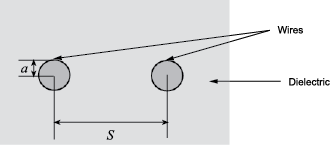# txlineTwoWire

Create two-wire transmission line

## Description

Use the `txlineTwoWire` object to create a two-wire transmission line. A cross-section of a two-wire transmission line is shown in this figure. The physical characteristics of a two-wire transmission line include the radii of the conducting wires, a, the separation or physical distance between the wire centers, S, and the relative permittivity and permeability of the wires. RF Toolbox™ software assumes that the relative permittivity and permeability are uniform.## Creation

### Syntax

``twowiretxline = txlineTwoWire``
``twowiretxline = txlineTwoWire(Name,Value)``

### Description

````twowiretxline = txlineTwoWire` creates a default two-wire transmission line object.```

example

````twowiretxline = txlineTwoWire(Name,Value)` sets Properties using one or more name-value pairs. For example, ```txline = txlineTwoWire('Separation',0.0046)``` creates a two-wire transmission line with a dielectric thickness of `0.0046` meters.```

## Properties

expand all

Name of the two-wire transmission line, specified as a string scalar or a character vector.

Example: `'Name','twowire1'`

Example: `twowiretxline.Name = 'twowire1'`

Data Types: `char` | `string`

Physical length of the transmission line, specified as a positive scalar in meters.

Example: `'LineLength',0.0200`

Example: `twowiretxline.LineLength = 0.0200`

Data Types: `double`

Conducting wire radius in the two-wire transmission line, specified as a positive scalar in meters.

Example: `'Radius',0.000970`

Example: `twowiretxline.Radius = 0.000970`

Data Types: `double`

Thickness of the dielectric, specified as a positive scalar in meters.

Example: `'Separation',0.0025`

Example: `twowiretxline.Separation = 0.0025`

Data Types: `double`

Relative permeability of the dielectric, specified as a positive scalar. Relative permeability of the dielectric, μ, to the permeability in free space, μ0.

Example: `'MuR',1.5`

Example: `twowiretxline.MuR = 1.5`

Data Types: `double`

Relative permittivity of the dielectric, specified as a positive scalar.

Example: `'EpsilonR',3.3`

Example: `twowiretxline.EpsilonR = 3.3`

Data Types: `double`

Loss angle tangent of the dielectric, specified as a nonnegative scalar in degrees

Example: `'LossTangent',1`

Example: `twowiretxline.LossTangent = 1`

Data Types: `double`

Conductivity of the conductor, specified as a scalar in Siemens per meter (S/m).

Example: `'SigmaCond',2`

Example: `twowiretxline.SigmaCond = 2`

Data Types: `double`

Stub transmission line termination, specified as `'NotApplicable'`, `'Open'` or `'Short'`.

Example: `'Termination','Short'`

Example: `twowiretxline.Termination = 'Short'`

Data Types: `char`

Type of stub, specified as `'NotAStub'`, `'Series'` or `'Shunt'`.

Example: `'StubMode','Series'`

Example: `twowiretxline.StubMode = 'Series'`

Data Types: `char`

Number of input and output ports, specified as a positive scalar.

Data Types: `double`

Terminals of coaxial transmission line, specified as a cell array of strings.

Data Types: `char` | `string`

## Object Functions

 `sparameters` Calculate S-parameters for RF data, network, circuit, and matching network objects `groupdelay` Group delay of S-parameter object or RF filter object or RF Toolbox circuit object `noisefigure` Calculate noise figure of transmission lines, series RLC, and shunt RLC circuits `getZ0` Calculate characteristic impedance with and without dispersion for transmission line `circuit` Circuit object `clone` Create copy of existing circuit element or circuit object

## Examples

collapse all

Create a two-wire transmission line using these specifications:

• Dielectric - air

• Thickness of dielectric or separation - 1.088 mm

• Permittivity or `EpsilonR` - 1.0054

`twowiretxline = txlineTwoWire('Radius',0.5e-3,'EpsilonR',1.0054,'Separation',1.088e-3);`

Calculate the noise figure and the group delay of the transmission line at 2.5 GHz.

`nf = noisefigure(twowiretxline,2.5e9)`
```nf = 0 ```
`gd = groupdelay(twowiretxline,2.5e9)`
```gd = 3.3446e-11 ```